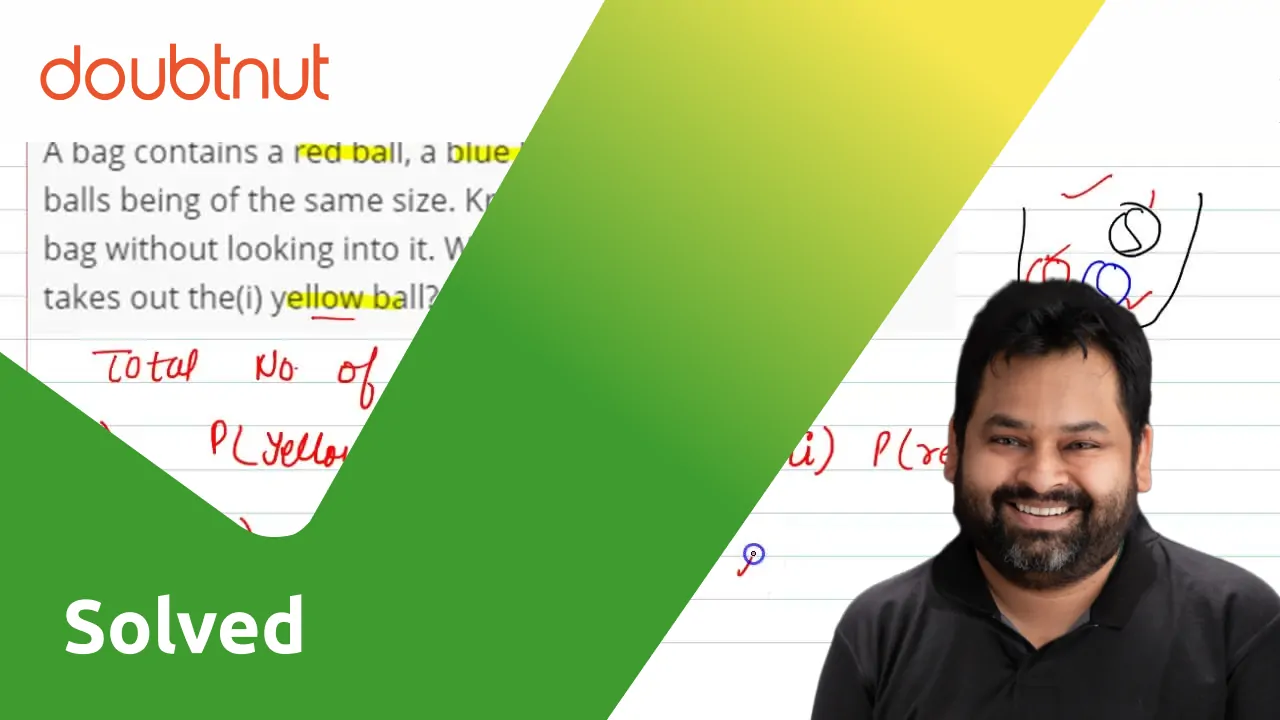Doubtnut App - Get 50 Lakh+ video solutions better than GoogleInstall Now

# A bag contains a red ball, a blue ball and a yellow ball, all the balls being of the same size. Kritika takes out a ball from the bag without looking into it. What is the probability that she takes out the(i) yellow ball? (ii) red ball?     (iii) blue ball?

Text Solution
Verified by Experts

## As there are total three balls of different colors in the bag. So, n(S)=3 So, probability Kritika takes out yellow ball, P(Y)=13 Similarly, probability Kritika takes out red ball, P(R)=13 Probability Kritika takes out blue ball, P(B)=13